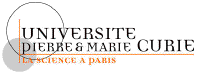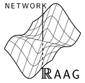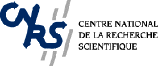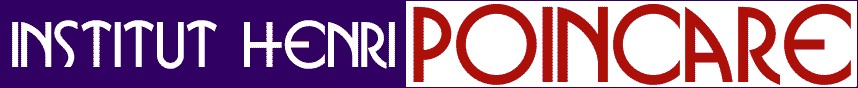# TRIMESTER ON REAL GEOMETRY September 12th – December 16th, 2005

## Abstracts of the courses

• F. Acquistapace (September 14th - October 13th, 8 lectures): Around Hilbert's 17th problem for analytic functions

In this course we will study several properties of the ring of analytic functions on a real analytic manifold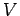. We will also study important properties of the sets which are defined using the ring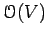of analytic functions on.
We will discuss several problems for those global functions and sets, which are classical for semialgebraic sets and regular functions, namely:
•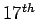Hilbert Problem: Let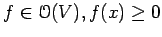for any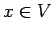. Is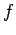a sum of squares of meromorphic functions?
• Nullstellensatz: Given an ideal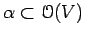, how can we caracterize the ideal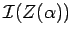?
• Positivstellensatz: Given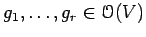, how can we caracterize the set of functions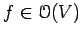which are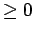on the set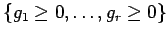?
• Closure: Is the closure of a global analytic set still a global semianalytic set?
• Connected components: Is a union of connected components of a global semianalytic set still a global semianalytic set?
• Finiteness: Let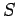be a global open (resp. closed) semianalytic set. Canbe described as a finite union of basic open (resp. basic closed) global semianalytic sets?
Here basic open (resp. basic closed) sets means sets of the form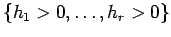(resp.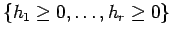), where each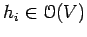.
We point out that almost all these problems are still open in the general case, for dimension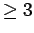.
We will start by showing the relations between the solution of theHilbert problem and the set of orderings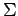of the field of fractions or of the ring of functions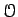. The crucial point here is the so called Artin-Lang Property which relates, roughly speaking, the sets which are definable by, that is, the global semianalytic sets, with the constructible sets of orderings of.

We will see that this property holds always for dimension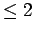and for a compact manifold. As an application, we will show how to solve Hilbert problem and to prove that the closure and the connected components of a global semianalytic set in a smooth analytic surface are still global semianalytic sets.
The Artin-Lang Property is not known for non compact manifolds of dimension. However, one can solve, for instance, the finiteness problem using more traditional tools, as sheaf theory, Cartan's Theorem B, Whitney's approximation theorem. For the sake of the audience, we will recall briefly these classic technics.
At the end of the course,we will present some (partial) recent results related to theHilbert Problem, the Nullstellensatz and the Positivestellensatz, some of which are still work in progress.

• S. Basu (November 16th  - 25th, 4 lectures): Efficient Algorithms for Computing the Betti Numbers of Semi-algebraic Sets.
Computing homological information of semi-algebraic sets (or more generally constructible sets) is an important problem for several reasons. From the point of view of computational complexity theory, it is the next logical step after the problem of deciding emptiness of such sets, which is the signature NP-complete problem in appropriate models of computation.
In this course  I will describe some recent progress in designing efficient algorithms for computing the Betti numbers of semi-algebraic sets in several different settings. I will describe a single exponential time algorithm for computing the first few Betti numbers in the general case and polynomial time algorithms in case the set is defined in terms of quadratic inequalities. One common theme underlying these algorithms is the use of certain spectral sequences -- namely, the Mayer-Vietoris spectral sequence and the cohomological descent'' spectral sequence first
introduced by Deligne.
Certain parts of this work  is joint with R. Pollack, M-F. Roy and (separately) with T. Zell.)

• F. Catanese (November 10th - 29th, 8 lectures) : Deformation types of real algebraic functions and manifolds
This course will focus on the complex point of view in the investigation of real algebraic varieties. This means that for instance the  real manifolds are viewed as the real part of a compact complex manifold, often projective or Kaehler, endowed with an antiholomorphic involution. And the point of view consists in (instead of forgetting the non real part) trying to see how the real part embeds into the complex manifold.

A first example of the advantage of taking  this point of view emerged historically even when  dealing with maximal real polynomials in 1 variable:  C. Davis proved with elementary real analysis that, given (n-1)  real critical values, there is a real  polynomial of degree n with these critical values. Later Thom observed how these kind of results are a consequence of Riemann's existence theorem, which sheds light more generally on the classification of real algebraic functions. In fact, once the real critical value sets are fixed, real algebraic functions are then seen just as monodromies which are invariant by complex conjugation on the complex line. I will focus  here on the least complicated examples, such as the counting of Arnold's so-called "snake sequences", leading to an easy generating function.

Another very easy concept which will be introduced pretty soon is the concept of the real (orbifold)-fundamental group of a real manifold: this group contains the complex fundamental group as a subgroup of index two, and it splits as a semidirect product if there are real points (observe that from the complex point of view also the empty set is interesting, as one can easily argue considering the mathematical charm of the Klein bottle). The use of this concept will be illustrated by the first important issue, which is the treatment of real structures on complex tori. Particular enphasis will be set on studying real elliptic curves and their deformations. This leads to an easy but important understanding of the genus 1 case of the relation between moduli spaces of real curves, and the real part of the moduli space of curves. This relation will then also be treated, even if sketchily, for the higher genus case. The topic of Nielsen Teichmueller realization, treated here, will show up and prove quite important also later.

Turning to higher dimensions, and in the Kaehler case, Hodge theory plays an important role, as was shown by Kharlamov in the 70's, in order to give topological estimates for Betti numbers of real manifolds.

Another  simple application of Hodge theory concerns the existence of irrational pencils, a theme which in turns leads to surfaces isogenous to a product and to those among them which are rigid: the so-called Beauville surfaces. These surfaces are rather important because they provide interesting examples of complex surfaces which do not admit real structures, yet can be isomorphic to their complex conjugate surface. The question of reality or non reality of rigid surfaces is not  yet fully investigated: I will also briefly discuss the interest of such surfaces  concerning the action of the absolute Galois group, in close relation to Grothendiecks' program called 'Dessins d' enfants'.

I will then briefly outline the Enriques-Kodaira classification of complex algebraic surfaces, and  the Kodaira classification of elliptic fibrations. I will then review the status of the  Enriques-Kodaira classification of real algebraic surfaces, through the contributions of several authors,  and with diverse methods. In particular, I will try to introduce to some important open problems about real surfaces of special type.  One such instance is the notion of quasi-simplicity considered by Degtyarev, Itenberg and Kharlamov , which revolves around the problem of comparing deformation and differentiable equivalence for real structures on complex algebraic surfaces.

• G. Comte (October 4th - 26th, 8 lectures): Propriétés métriques en géométrie modérée

Measures on Grassmannians,
Hausdorff and entropy dimensions,
Vitushkin variations and Lipschitz-Killing curvatures,
Critical locus and entropy,
Semialgebraic complexity of applications,
Quantitative Sard theorems,
Localizations of invariants of integral geometry in tame geometry,
Conormal cone, normal cone and regularity conditions,
Variations of local invariants along regular strata.

• M. Coste (September 13th - 28th, 8 lectures) : Ensembles constructibles en géométrie réelle ; géométrie modérée

I shall give in this course the basics of semialgebraic geometry and tame geometry (o-minimal structures) for students who are not familiar with these topics, in order that they can follow more specialized courses of the trimester. I shall review the cylindrical decomposition into cells, stratifications,  triangulation, the local conic structure, Hardt's trivialization theorem, growth properties of semialgebraic functions. I shall insist on uniformity results for semialgebraic (or definable) families.

• M. Dickmann (September 13th - October 24th, 8 lectures) : Théorie des modèles en algèbre et géométrie

Notions et results of model theory necessary for the proof of quantifier elimination for real closed fields and related results.
Application of these tools to:
• the structure of semialgebraic sets and functions,
• the real Nullstellensatz and the Artin-Lang theorem,
• simple zeros and positivity criteria for polynomials,
• topology of semialgebraic sets: cellular decomposition, dimension, Thom's lemma, triviality of semialgebraic families,
• theory of the real spectrum; real spectrum of varieties,
• continuous semialgebraic functions and Nash functions, Lojasiewicz inequality, Artin's approximation theorem,
• commutative algebra of Nash functions.

• L. van den Dries (November 28th - December 14th, 8 lectures): Asymptotic differential algebra

This is differential algebra in a setting where there is an ordering and valuation compatible with the derivation, as in Hardy fields, fields of transseries and H-fields.

• A. Gabrielov (October 20th - 28th, 4 lectures): Pfaffian functions and sparsity. Real Schubert Calculus and the B. and M. Shapiro Conjecture
• Lecture 1. Complexity of computations with Pfaffian functions
Pfaffian functions introduced by Khovanskii in 1970-ies have polynomial-like global finiteness properties. These functions satisfy a triangular system of Pfaffian (first order partial differential) equations with polynomial coefficients. Khovanskii-Bezout theorem gives an upper bound on the number of real solutions to systems of Pfaffian equations in terms of the number of Pfaffian equations and the degrees of polynomials in the definition of Pfaffian functions. Important special cases of Pfaffian functions are fewnomials, i.e., polynomials with few nonzero monomials. Complexity of fewnomials as Pfaffian functions depends on the number of monomials, not on their degrees.
A review of the upper bounds on the complexity of computations with Pfaffian functions and semi-Pfaffian sets will be given, with the special attention to fewnomial semialgebraic sets.
• Lecture 2. Betti numbers of sets defined by formulas with quantifiers
A spectral sequence associated with a surjective mapping allows one to provide upper bounds for the Betti numbers of a wide class of sets defined by formulas with quantifiers in terms of the Betti numbers of auxiliary sets defined by quantifier-free formulas. In the ordinary semialgebraic case, this approach provides better upper bounds than the quantifier elimination, especially for the sparse polynomials. It works also for Pfaffian functions.
• Lecture 3. Rational functions with real critical points, the Catalan numbers, and the Schubert calculus
How many rational functions of degree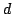have a given set of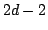points as their set of critical points? If we identify functions that differ by a fractional-linear transformation in the target space (all such functions have the same critical points) the answer is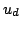, the Catalan number.
This is equivalent to a problem in the Schubert calculus: How many codimension 2 affine subspaces in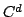intersect affine lines tangent to the rational normal curve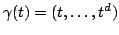atdistinct points? The answer (Schubert, 1886) is, the Catalan number.
Theorem. Suppose that allpoints are real. Then allequivalence classes of rational functions with these critical points are real (contain real functions).
The corresponding result in the Schubert calculus is a special case of the B. and M. Shapiro conjecture.
More general problem: Givennon-overlapping real segments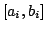, how many rational functions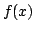of degreesatisfy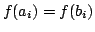for all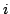?
The answer is again, the Catalan number, and all these functions (up to equivalence) are real.
In the Schubert calculus problem, one should replace tangents to the rational normal curve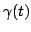by the secants through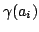and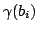. These secants become tangents when the segments contract to points.
If the segments do overlap, not all solutions are real, but there are lower bounds on the number of real solutions. These results are related to the B. and M. Shapiro conjecture for flag varieties.

• Lecture 4. Degree of the real Wronski map and the pole placement problem in control theory.
The Wronski map associates to a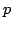-tuple of polynomials of degree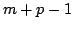their Wronski determinant, a polynomial of degree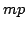. If the polynomials are linearly independent, they define a a point in the Grassmannian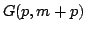. Accordingly, the Wronski map can be considered as a map fromto the projective space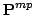. The map is finite, and one can define its degree. In the complex case, this degree equals the number of standard Young tableaux for the rectangular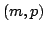-shape. In the real case, Young tableax should be counted with the signs depending on the number of inversions. Degree of the real Wronski map is zero when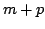is even, and equals the number of standard shifted Young tableaux for an appropriately defined shifted shape whenis odd. When both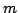andare even, the Wronski map is not surjective. These results have important applications to real Schubert Calculus and to the pole placement problem in control theory. In particular, non-surjectivity of the real Wronski map provides examples of linear systems with static output feedback for which the pole placement problem has no real solutions.

• L. González-Vega (October 20th - 26th, 4 lectures): Using real algebraic geometry to improve curve and surface algorithms in computer aided geometric design applications

By using the surface--to--surface intersection problem in Computer-Aided Geometric Design as motivation, it will be shown how techniques from Real Algebraic Geometry can be very helpful in order to improve in practice the computation of the intersection curve between two surfaces in 3D space.

The topics covered by the four lectures will be the following:
• Lecture 1: How curves and surfaces are represented and manipulated in Computer-Aided Geometric Design?
• Lecture 2: Solving intersection problems: dealing with real algebraic plane curves or surfaces implicitely defined.
• Lecture 3: Computing with offsets: detecting geometric extraneous components.
• Lecture 4: Interference characterization between conics and quadrics through Quantifier Elimination.

• S. Kuhlmann (September 26th - October 17th, 8 lectures) : Polynômes positifs et problèmes de moments

The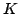-moment problem originates in Functional Analysis: for a linear functional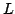on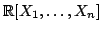, one studies the problem of representingvia integration. That is, one asks whether there exists a measure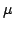, on Euclidean space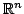, supported by some given (basic closed semi-algebraic) subsetof, such that for every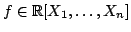we have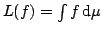. Via Haviland's Theorem, the-moment problem is closely connected to the problem of representing positive (semi)definite polynomials on(Hilbert's 17th Problem, Positivstellensatz). In his solution of the Moment Problem for compact, Schmüdgen (1991) exploits this connection, and proves that a surprisingly strong version of the Positivstellensatz holds in the compact case. Schmüdgen's result provides a strong motivation to study refined versions of the Positivstellensatz. Following rapidly on his work, several generalizations of his results were worked out. (See F. Acquistapace's course for related topics in the context of analytic functions). The aim of this course is to provide the beginning student with a brief account of these developments. More precisely, we plan to cover the following topics:
• Hilbert's 17th Problem,
• Stengle's Positivstellensatz,
• Schmüdgen's Positivstellensatz,
• applications to the multi-dimensional-moment problem,
• the moment problem for subsets of the real line,
• extending Schmüdgen's Theorem to non-compact sets.
If time permits, we will discuss a version of the-moment problem, whenis assumed to be invariant under the action of a group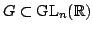. Throughout the course, we shall present open problems.

• A. Macintyre (October 17th - November 15th, 8 lectures): Model theory of elliptic functions

I will consider model-theoretic questions for the various structures (T,f), where T is a complex torus of dimension 1 and f is a corresponding Weierstrass elliptic function. Model-completeness results will be proved for each individual case, in a formulation where we consider the f as given by its real and imaginary parts, which enables us to bring into play various techniques from o-minimality. The attempt to get a uniform model-completeness is till under way, and seems to be of great difficulty and considerable interest. By work of Peterzil and Strachenko, the uniform theory is known to be o-minimal, because (nontrivially) interpretable in the theory of the restricted analytics and the global real exponential. I will discuss the fine detail of this,and lay out the obstructions to getting uniform model-completeness (these have to do with Manin's work, and differential equations for the periods).I go on to show how certain cases (T,f) are even decidable, modulo a conjecture of Andre on 1-motives and transcendence. I will explain the connection to the Wilkie-Macintyre proof of decidability for the real exponential using Schanuel's Conjecture.Finally, I will discuss what is presently known for related functions, e.g the zeta and sigma functions.

• L. Mahé (November 10th - December 16th, 12 lectures) : Formes quadratiques et géométrie réelle

Real algebraic (and semialgebraic) geometry deals with sets of real solutions of polynomial equations (and inequalities), and the algebra underlying this geometry is the one of sums of squares, or more generally of quadratic forms. Thus the latter turn out to play a fundamental role. After some basics of semialgebraic geometry (real closed fields, semialgebraic sets), real algebra  (Nullstellensatz and Positivstellensatz, real spectrum), and quadratic forms (Pfister forms, Witt rings), the course will illustrate the interactions between geometry and quadratic forms with the study of the following three problems:
• bounding the number of squares intervening in sums of squares,
• bounding the number of inequalities needed to describe a semialgebraic set
• separating connected components of varieties by signatures of quadratic forms.
Bibliography:
1. J. BOCHNAK, M. COSTE, M.-F. ROY : Géométrie algébrique réelle, Springer (1987)
2. C. ANDRADAS, L. BRÔCKER, J. RUIZ, Constructible sets in real geometry, Springer (1996)
3. T.-Y. LAM, The algebraic theory of quadratic forms, Reading, Benjamin (1973)
4. W. SCHARLAU, Quadratic and hermitian forms, Springer (1985)

• C. McCrory (September 28th - October 28th, 16 lectures) : Invariants and singularities

In the first half of the course I will discuss Akbulut-King invariants, which are local topological obstructions for a semialgebraic set to be homeomorphic to a real algebraic set. I'll present work with Adam Parusinski defining these invariants using operators on the ring of constructible functions. as well as related work by Coste-Kurdyka and Akbulut-King. The second half of the course will be on virtual Betti numbers, which are global invariants of real algebraic varieties that Parusinski and I introduced. I'll explain their relation to the weight filtration of Totaro and to the work of Bittner and Guillen-Navarro. Throughout the course I will emphasize open problems.

• G. Mikhalkin (September 23rd - December 16th, 16 lectures) : Amoebas of algebraic varieties and tropical geometry

This course is intended to introduce the audience to a recent technique in Algebraic Geometry based on application of the moment map and toric degenerations. One of the simplest examples of the moment map is the logarithm map that takes a point of the complex torus C*n to the point in Rn obtained by taking the logarithm of the absolute value coordinatewise . The images of holomorphic subvarieties of C*n under this map are called amoebas.

If one modifies this moment map by taking the logarithm with base t and lets t to go to infinity then the amoebas tend to some piecewise-linear polyhedral complexes in Rn. The dimension of these limiting complexes is equal to the dimension of the original varities. It turns out that such complexes can be considered as algebraic varieties over the so-called tropical semifield. The term "tropical semifield" appeared in Computer Science and, in the current context, refers to the real numbers augmented with the negative infinity and equipped with two operations, taking the maximum for addition and addition for multiplication. Polynomials over the tropical semifield are convex piecewise-linear functions and geometric objects associated to these polynomials are certain piecewise-linear complexes in Rn.

In the course we consider applications of both the amoebas themselves and the resulting tropical geometry. One area where amoebas turn out to be useful is Topology of Real Algebraic Varieties, in particular, problems related to Hilbert's 16th problem. Using amoebas we show topological uniqueness of a homologically maximal curve in the real torus R*2 and deduce a partial topological description for hypersurfaces in R*n for n>2. Applications of tropical geometry include construction of real algebraic varieties with prescribed topology (patchworking) as well as enumerative geometry.

A typical problem in enumerative algebraic geometry is to compute the number of curves of given degree and genus and with a given set of geometric constraints (e.g. passing through a point or another algebraic cycle, being tangent to such cycle, etc.). For a proper number of geometric constraints one expects a finite number of such curves. Even in the cases when this number is not finite there exists a way to interpret the answer to such problem as a (perhaps fractional or negative) Gromov-Witten number. Tropical geometry can be used for computation of these numbers. In this course we'll compute such numbers for arbitrary genus and degree when the ambient space is a toric surface and for genus 0 (and arbitrary degree) if the ambient space is a higher-dimensional toric variety. In addition we consider real counterparts of the enumerative problems, in particular, the Welschinger invariant, and do some computations for them.

• S. Orevkov (September 15th - October 7th, 8 lectures) : Courbes algébriques réelles, tresses et courbes J-holomorphes

• P. Parrilo (November 21st - 24th, 4 lectures): Computational techniques based on sum of squares decompositions

The idea would be to cover the application of convex optimization methods to problems in real algebraic geometry. We'd start with the basic notions of  semidefinite programming, and SOS decompositions. Depending on the audience, we may go into SOS on quotient or invariants rings, and apply the results to the computation of Positivstellensatz certificates.
Also, again depending on the audience, we would emphasize different kind of applications (geometry, dynamical systems, quantum mechanics, etc.)

• L. Paunescu (December 1st - 16th, 4 lectures), Tree Model, Relative Newton Polygon and Applications

The Newton Polygon is a powerful tool for analytic singularities. It yields the fundamental theorem of Newton-Puiseux. This is an elementary exposé on this.
For an analytic function germ (convergent power series)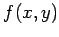and an analytic arc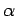, the Newton Polygon ofrelative to,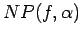is defined. A number of important applications are illustrated.
Two seemingly unrelated problems are intimately connected.
The first is the equisingularity problem in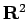: For an analytic family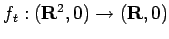, when should it be called an equisingular deformation? This amounts to finding a suitable trivialization condition (as strong as possible) and, of course, a criterion.
The second is on the Morse stability. We define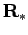, which is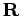"enriched" with a class of infinitesimals. How to generalize the Morse Stability Theorem to polynomials over?
The spaceis much smaller than the space used in Non-standard Analysis. Our infinitesimals are analytic arcs, represented by fractional power series, e.g.,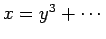,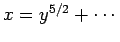,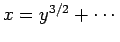, are infinitesimals at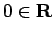, in descending orders.
Thus,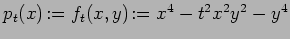is a family of polynomials over. This family is not Morse stable:a triple critical point insplits into three when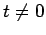.
Bibliography:
1. T.-C. Kuo and A. Parusinski, Newton Polygon Relative to an Arc, in Real and Complex Singularities (São Carlos, 1998), Chapman & Hall Res. Notes Math., 412, 2000, 76-93.
2. T.-C. Kuo and A. Parusinski, Newton-Puiseux Roots of Jacobian Determinants, Journal of Algebraic Geometry, 13 (2004), 579-601.
3. K. Kurdyka and L. Paunescu, Arc-analytic Roots of Analytic Functions are Lipschitz, Proceedings of the American Mathematical Society, 13 (2004), no. 6, 1693-1702.
4. T.-C. Kuo and L. Paunescu, Equisingularity inas Morse stability in infinitesimal calculus, to appear in the Proceedings of the Japan Academy, June, 2005.

• J.-Ph. Rolin (November 16th - December 15th, 8 lectures) : La o-minimalité du point de vue de la géométrie et de l’analyse

This course is the continuation of M. Coste's one. We will try to answer, in concrete situations, the question : How to prove that a given family of sets is o-minimal ?
More precisely, the topics considered will be :

• the properties of global sub-analytic sets, and a preparation theorem for sub-analytic functions
•  the exp-log-analytic functions
• the behavior of solutions of differential equations from the o-minimal point of view
• the relationship with the notion of quasi-anayticity

• F. Rouillier (September 14th - 28th, 5 lectures), M.-F. Roy (October 3rd - 12th, 5 lectures), S. Basu (November 14th - November 28th, 5 lectures) : Algorithms in real algebraic geometry
• F. Rouillier:
1. Univariate solving.
2. Properties and applications of Gröbner bases (localization and elimination).
3. Zero-dimensional solving (Hermite quadratic forms, RUR)
4. Well-behaved parametric systems
5. Some applications of polynomial system solving
• M-F. Roy:
1. Discriminants, Resultants, Subresultants
2. Complexity of cylindrical decomposition
3. Applications of cylindrical decomposition
4. Complexity of finding a point in every connected components of an algebraic set
5. Complexity of finding non empty sign conditions on a family of polynomials
• S. Basu:
1. General Decision Problem and Quantifier Elimination.
2. Uniform (Local) Quantifier Elimination and its application in constraint databases.
3. Computing Roadmaps for Algebraic Sets.
4. Computing Roadmaps in general and computing descriptions of connected components.
5. Computing coverings by contractible sets and applications.

• E. Shustin (September 29th - October 14th, 4 lectures): Patchworking construction and its applications

1. Construction of real non-singular algebraic varieties: Viro's method and its modifications.
2. Patchworking construction in singularity theory and algebra over the complex and real field.
3. Patchworking construction in the tropical geometry.

• F. Sottile (November 14th - 29th, 8 lectures) : Real solutions to equations from geometry

Understanding, finding, or even deciding the existence of real solutions to a system of equations is a very difficult problem with many applications. While it is hopeless to expect much in general, we know a surprising amount about these questions for systems which possess additional structure. Particularly fruitful---both for information on real solutions and for applicability---are systems whose additional structure comes from geometry. Such equations from geometry for which we have information about their real solutions will be the subject of this short course.

We will focus on equations from toric varieties and homogeneous spaces, particularly Grassmannians. Not only is much known in these cases, but they encompass some of the most common applications. The results we discuss may be grouped into three themes:
(1) Upper bounds on the number of real solutions.
(2) Geometric problems that can have all solutions be real.
(3) Lower bounds on the number of real solutions
Upper bounds as in (1) bound the complexity of the set of real solutions---they are one of the sources for the theory of o-minimal structures which are an important topic in this trimester. Lower bounds as in (3) give an existence proof for real solutions. Their most spectacular manifestation is the non-triviality of the Welschinger invariant, which was computed via tropical geometry. This is also explained in other courses this trimester at the Centre Borel.

The course will have three parts, grouped by geometry:

I) Overview (Lecture 1)
II) Toric Varieties (Lectures 2--4)
III) Grassmannians (Lectures 5--8)

Topics for each lecture
1. Overview. Upper and lower bounds, Shapiro conjecture, and rational curves interpolating points in the plane.
2. Sparse polynomial systems and toric varieties. Kouchnirenko's Theorem and Groebner degeneration.
3. Upper bounds. Descartes' rule of signs, Khovanski's fewnomial bound, bound for circuits.
4. Lower bounds. Soprunova-Sottile toric lower bound.
5. Grassmannians. Wronski map, problem of 4 lines. Reality in the Schubert calculus via Schubert induction, Vakil's Theorem.
6. The Shapiro conjecture for Grassmannians. Computational and theoretical evidence. Sums of squares and discriminants.
7. Eremenko and Gabrielov's elementary proof of Shapiro Conjecture for 2-planes. Maximally inflected curves.
8. Lower bounds for the Wronski map via sagbi degeneration.

There will be typeset notes available for each lecture.

• B. Teissier (September 19th - October 17th, 5 lectures) : Introduction to valuations in algebraic geometry
Je donnerai les bases de la theorie des valuations en insistant sur leur role en geometrie algébrique. J'essaierai de rappeler et d'illustrer les notions de géométrie algébrique utilisées pour les rendre accessibles aux non-experts.
I will explain the basics of the theory of valuations, insisting on their role in algebraic geometry. I shall try to recall and illustrate the notions of algebraic geometry which are used, in order to make them accessible to non-experts.

D. Gondard (October 24th - November 14th, 3 lectures) : Valuations in real algebra
Le cours, qui sera effectué dans le cadre des corps ordonnables,  débutera avec la compatibilité d'un préordre et d'une valuation, et les  cas particuliers des éventails et éventails valués. Ensuite nous présenterons la théorie des ordres de niveau supérieur, et ses liens avec les sommes de puissances ; puis il sera etudié la notion de clôture algébrique d'un corps muni d'un éventail valué. Enfin nous donnerons quelques applications des ces notions en géométrie algébrique réelle et montrerons le lien avec les R-places et l'anneau d'holomorphie réel.
We shall work in the framework of real fields, start with the compatibility of a preordering with a valuation, and then turn to the special case of fans and valuation fans. Afterwards we shall present the higher level orderings, and their links with sums of powers, and study the notion of algebraic closure under algebraic extensions of a fields equipped with a valuation fan. Last we shall give some applications to Real Algebraic Geometry and show the links with R-places and with the real holomorphy ring.